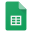Remote Plans (10)

Reading - First - Fourth Quarters

Grade Level Text: Q 1 = Level J, Q 2 = Level L, Q 3 = Level L, Q 4 = Level M.

*Students will develop accuracy, phrasing, and expression while reading a variety of grade-level print/digital text to support comprehension.

*Students will construct meaning by using prior knowledge and text information while reading grade-level literary and informational text.

Math: First Quarter:

*Students will compute addition and subtraction to and from 20.

*Students will fluently add whole numbers to 20 (0,1,2)

*Students will fluently subtract whole numbers from 20 (0,1,2

*Students will solve story problems - adding to, subtracting from, joining.

*Students will demonstrate multiplication using these methods: array, repeated addition, equal groups, open number line.

Science: First Quarter: Interdependent Relationships in Ecosystems (Plants and Animals)

Math: Second Quarter:

First Quarter Learnings with these additions:

*Students will fluently add whole numbers to 20 (doubles, making 10)

*Students will fluently subtract whole numbers from 20 (doubles, making 10)

*Students will solve story problems - adding to, subtracting from, joining, separating, comparing, array (5x5 or less).

*Students will compute addition- 2 digit: add within 100 using strategies based on place value, including the standard algorithm, properties of operations, and/or the relationship between addition and subtraction, using concrete models, drawings, and strategies, which reflect understanding of place value and properties of operations.

*Students will demonstrate place value within 100: comparing numbers using symbols, reading and writing numbers within the range of 0-100 using standard, word, and expanded forms, count in groups of 5s, 10s, and 100

Math: Third Quarter:

First & Second Q Learnings with these additions:

*Students will fluently add whole numbers to 20 (using doubles, adding 10)

*Students will fluently subtract whole numbers from 20 (using doubles, adding 10)

*Students will compute addition and subtraction:

3 digit up to and within 1000 using strategies based on place value, including the standard algorithm,

properties of operations, and/or the relationship between addition and subtraction, using concrete models, drawings, and strategies, which reflect understanding of place value and properties of operations.

*Students will demonstrate place value within 1000: comparing numbers using symbols, reading and writing numbers within the range of 0-1000 using standard, word, and expanded forms, count in groups of 5s, 10s, and 100s.

Story Problems - adding to, subtracting from, joining, separating, array, unknown, two step, story problems within 100.

Science : Third Quarter: Earth's Systems: Processes that Shape the Earth (Landforms)

Math: Fourth Quarter

First -Third Quarter Learnings with these additions:

*Students will fluently add whole numbers to 20 (using tens)

*Students will fluently subtract whole numbers from 20 (using tens)

* Students will demonstrate understanding of money to \$1.00 using mixed coins.

* Students will demonstrate understanding of time to 5 minutes.

* Students will measure length (feet, inch, meter, centimeter, yard).

*Students will demonstrate Geometry including: solid figures, plane shapes up to hexagons, fractions, area with square units, and splitting shapes into equal parts.

Science: Fourth Quarter: Structure and Properties of Matter